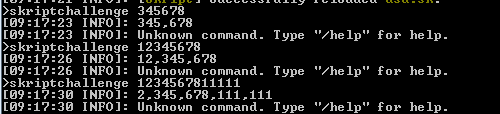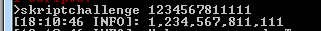# [#1] Skript Challenge - Number Format

## Do you like Skript Challenges like this?

• ### No

• Total voters
52
• Poll closed .
Status
Not open for further replies.
code_language.skript:
``````command /skriptchallenge [<text>]:
trigger:
if arg-1 is set:
set {_number::*} to arg-1 split at ""
loop size of {_number::*} times:
add last element of {_number::*} to {_number2::*}
set {_last} to size of {_number::*}
delete {_number::%{_last}%}

set {_time} to 1
set {_n} to -1
set {_integer} to ""
loop {_number2::*}:
set {_integer} to "%loop-value%%{_integer}%"
if ({_n} / 3) = {_time}:
if {_n} + 1 isn't size of {_number2::*}:
set {_integer} to ",%{_integer}%"

send "%{_integer}%"
stop``````Last edited by a moderator:
code_language.skript:
``````command /skriptchallenge [<text>]:
trigger:
if arg-1 is set:
set {_number::*} to arg-1 split at ""
loop size of {_number::*} times:
add last element of {_number::*} to {_number2::*}
remove last element of {_number::*} from {_number::*}

set {_time} to 1
set {_n} to -1
set {_integer} to ""
loop {_number2::*}:
set {_integer} to "%loop-value%%{_integer}%"
if ({_n} / 3) = {_time}:
if {_n} + 1 isn't size of {_number2::*}:
set {_integer} to ",%{_integer}%"

send "%{_integer}%"``````
View attachment 163
i count 5 1's on the end of that number, why's it returning 6 :O

i count 5 1's on the end of that number, why's it returning 6 :O
5 1's? - - what u mean

5 1's? - - what u mean
Why does "1234567811111"

return

2,345,678,111,111

it should return

1,234,567,811,111

No one followed this rule except me or I didn't get it.
I knowCode:
``````function a(b: text) :: text:
if length of {_b} > 3:
return "%a(first length of {_b} - 3 characters of {_b})%,%last 3 characters of {_b}%"
return {_b}

command /c <integer>:
trigger:
send a("%arg%")``````

Not sure whether the command was required, but it's there.

EDIT: Fixed the code formatting issue.
I'm not that much good at math specially when i try to make it in english.. because i'm not english so, i might use your code in some of my skripts (if you don't mind) and i will try to understand it moreGood work

•Tlatoani
Code:
``regex replace "(?<=\d)(?=(\d{3})+(?!\d))" with "," in {_integer}``
1 less characterCode:
``regex replace "(?<=\d)(?=(\d{3})+(?!\d))" with "," in {_integer}``
1 less characterThat's just using regex. Not really using the ability of Skript in any way. I don't really think that the challenge was about that, but it works.

That's just using regex. Not really using the ability of Skript in any way. I don't really think that the challenge was about that, but it works.
This one was about the shortest amount of code. I will however be making some focused on code design instead of character count. Those ones I expect will show a completely different type of answer.

No one followed this rule except me or I didn't get it.

Why does "1234567811111"

return

2,345,678,111,111

it should return

1,234,567,811,111Edited. now work

Got in here with high hopes, hoping I've learned something and that I'd be able to join this challenge... saw numbers, saw math, headache hit, too dumb for this. GG, must admit, every code I saw (especially Snow-Pyon's(cuz shortest)) was pretty damn good. Anyone wants to teach me how to math?

Got in here with high hopes, hoping I've learned something and that I'd be able to join this challenge... saw numbers, saw math, headache hit, too dumb for this. GG, must admit, every code I saw (especially Snow-Pyon's(cuz shortest)) was pretty damn good. Anyone wants to teach me how to math?
First you start doing math. Realise it's stupid, and stop.

That's how.

First you start doing math. Realise it's stupid, and stop.

That's how.

You don't really need that much math, just the modulus operator. All you need to do is do a reverse iteration through every char in the string, and when the char you're on is evenly divisible by 3 (I.E. char mod 3 = 0), add a comma.

An example in Java would be:
Code:
``````    public static String addCommas(String s){
String ret = "";
for(int i = s.length() - 1, j = 1; i > -1; i--, j++){//reverse iteration
ret = s.charAt(i) + ret;
if (j % 3 == 0){ //if evenly divisble by 3
ret = "," + ret;
}
}
return ret;
}``````

I would hardly call it difficult, Should be able to do this within your first year of taking a programming course.

Doing this in skript would be annoying because it doesn't have for loops, but it can be done:

Code:
``````//assume {s} is the string
{_ret} = ""
{_i} = {s}'s length
{_j} = 1
while {_i} > 0:
ret = text of {s} from i to i + {_ret}
if j mod 3 = 0:
ret = "'" + {_ret}
{_i} = i - 1
{_j} = j + 1
{s} = {_ret}
#Keep going``````

I haven't actually tested the above skript code, but if I didn't make any mistakes and the documentation is correct, it should work.

Last edited by a moderator:

If you want to call basic arithmetic a lot of math, then sure, I guess...

If you want to call basic arithmetic a lot of math, then sure, I guess...
He's not calling it a lot of math. He was only responding to you stating that you don't need a lot of math, just the modulus operator, while the modulus operator is math.

He's not calling it a lot of math. He was only responding to you stating that you don't need a lot of math, just the modulus operator, while the modulus operator is math.
That's why I said "You don't really need that much math, just the modulus operator." One operation is not a lot of math, like I said.

Here's my code, with no addons :
code_language.skript:
``````set {_r} to "%{_number}%"
set {_t} to ""
loop round up length of {_r} / 3 times:
set {_l} to the last 3 characters of {_r}
set {_r} to the first length of {_r} - 3 characters of {_r}
if loop-number = 1:
set {_t} to "%{_l}%%{_t}%"
else:
set {_t} to "%{_l}%,%{_t}%"``````

Formatted number will be in {_t}

Entries close on Monday, at whatever time I get up. I'll try and find the shortest answer but you lot argued about maths so much, I have no idea.

Status
Not open for further replies.